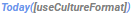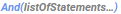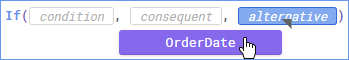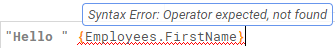## Formula Editor

The Formula Editor has a suite of features to help guide you when using formulas.

You can use the Search field to search through the functions by name. Or start typing in the Formula field to get a list of functions that match the text.List of functions that match the text

Tip
You can search for data fields, report cells, and parameters in the same manner.

# Using functions

Formulas work by applying some calculations to a few values that you give them. A basic example of a formula is 1 + 2. In this example, the formula comprises one function, the addition function (+), and two arguments, the numbers 1 and 2. When the report runs, the formula calculates and returns a value of 3.

Not every function takes two arguments, so functions cannot always be written as (argument1 function argument2). In most cases functions instead use the following format:

`Function(argument1, argument2, ...)`

The addition example could also be written as Add(1, 2). This is the style that most formulas in the application use. Most functions are more abstract than simple arithmetic. Each function has a description which tells you exactly what it does and how to use it.

## Arguments

An argument is a value that a function uses to do a calculation. Functions have different amounts and types of arguments. When a function is first entered into the Formula field, there are placeholder values for each required argument. Click on a placeholder to see the description of the argument.Description of the condition argument of the If function

Some arguments are optional. Those are surrounded by brackets [ ]. Some arguments are a list of values. Those are followed by an ellipsis (...).The Today function takes an optional argument. The And function takes a list of arguments.

Tip
Some functions take no arguments. These are formatted with empty parentheses:
`Function()`

You need to supply values for all of the function's required arguments. Type a value into the argument space, or drag a data field or function over the placeholder.Dragging a data field to an argument

Click OK when you are finished.

Formulas are used in several areas besides the report design: custom sorts and groups, drilldowns, and conditional formatting for cells and charts, to name a few. In every area where you can use a formula you can click on a formula icon to open a Formula Editor window. These areas may require a specific type of data to be returned from the formula. Some built-in functions, such as aggregates, may be unavailable. Consult the relevant topic for the specifics.

Formulas which reference data fields or cells, with the exception of aggregate functions, should be in the same report section as the reference data. Detail sections repeat for every data field, group sections repeat for every group, and page sections repeat for every page. Since most formulas expect only one reference value, and not repeated values, referencing a repeated field or cell from outside of its section can return irregular data. Formulas need to repeat alongside their reference values. Aggregate functions are the exception since they are designed to evaluate once for a group of data. For more information, see Sections.

# Manually typing formulas

You have the option of typing in your functions, data fields, parameters, and cell references manually. Use the following formatting guidelines.

### Text

Surround text with double or single quotation marks:

"Hello, World!"

'I am on fire'

If you want to use a quotation mark in the text, then surround the text with the opposite mark:

"You're on fire"

'He says "like" too often'

Do not use quotation marks around numbers.

### Data fields

{DataCategory.DataField}

where DataCategory is the name of the data category

and DataField is the name of the data field

### Cell references

To use the value from another cell in a formula, use the following format:

[C#]

where C is the letter of the cell column

and # is the number of the cell row

Caution
Rearranging cells can cause cell references to break.

### Parameters

Parameters return special values depending on some condition. The built-in parameters are:

• @pageNumber@ gives the current page of the report
• @reportName@ gives the name of the report
• @reportFullName@ gives the name and path to the report